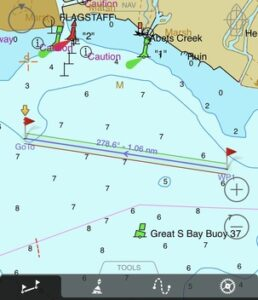# speed-or-velocity## 30 Sep speed-or-velocity“Spending time with my son this past week on a math project brings back 30+ year old flashbacks of doing the same with my Dad, “the PacMan of physics,” we called him. The “Physics” remains unchanged.

“Speed” vs. “Velocity”

Many people use the terms interchangeably but their difference is fundamental to math and physics principle equations.  Speed is a measurement, e.g. a speedometer gives you mile/hour that you car is moving.

Velocity on the other hand is what we use to describe movement in relation to time and distance.

In this case, distance from one position to another i.e. 278.6 deg

Velocity is a “vector” or mathematical variable depicted with a line/arrow representing direction.
V-> for velocity = distance/unit time.

Velocity is the derivative of acceleration, and foundation for the mathematics of motion. Give your “speed” direction in balance and proper form  Always, pjp